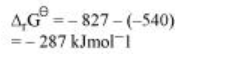# The value ofQuestion:

The value of ${ }_{\mathrm{l}} \mathrm{G}^{\theta}$ for formation of $\mathrm{Cr}_{2} \mathrm{O}_{3}$ is $-540 \mathrm{kJmol}^{-1}$ and that of $\mathrm{Al}_{2} \mathrm{O}_{3}$ is $-827 \mathrm{kJmol}^{-1} .$ Is the reduction of $\mathrm{Cr}_{2} \mathrm{O}_{3}$ possible with Al?

Solution:

The value of $\Delta_{1} \mathrm{G}^{\theta}$ for the formation of $\mathrm{Cr}_{2} \mathrm{O}_{3}$ from $\mathrm{Cr}\left(-540 \mathrm{kJmol}^{-1}\right)$ is higher than that of $\mathrm{Al}_{2} \mathrm{O}_{3}$ from $\mathrm{Al}\left(-827 \mathrm{kJmol}^{-1}\right)$. Therefore, $\mathrm{Al}$ can reduce $\mathrm{Cr}_{2} \mathrm{O}_{3}$ to $\mathrm{Cr}$. Hence, the reduction of $\mathrm{Cr}_{2} \mathrm{O}_{3}$ with $\mathrm{Al}$ is possible.

Alternatively,Subtracting equation (ii) from (i), we have

$2 \mathrm{Al}+\mathrm{Cr}_{2} \mathrm{O}_{3} \longrightarrow \mathrm{Al}_{2} \mathrm{O}_{3}+2 \mathrm{Cr}$As ${ }^{\Delta_{1} \mathrm{G}^{\theta}}$ for the reduction reaction of $\mathrm{Cr}_{2} \mathrm{O}_{3}$ by Al is negative, this reaction is possible.